Home > INT3 > Chapter 7 > Lesson 7.1.3 > Problem7-41

7-41.
1. Solve each of the following equations. Homework Help ✎

1. (x + 4)(2x – 5) = 0

2. (x + 4)(x2 – 5x + 6) = 0

3. 3x(x + 1)(2x – 7)(3x + 4)2(x – 13)(x + 7) = 0

4. Describe how to solve an equation made up of any number of factors whose product is zero.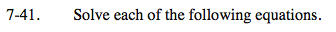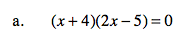Use the Zero Product Property.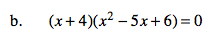Factor the quadratic expression and use the Zero Product Property.

$x = –4, 2,\text{ or } 3$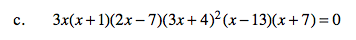Since the expression on the left is written as a product equal to zero, set each factor equal to zero and solve.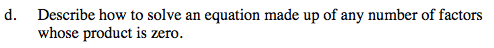What did you do to solve the equations in parts (a) through (c)?